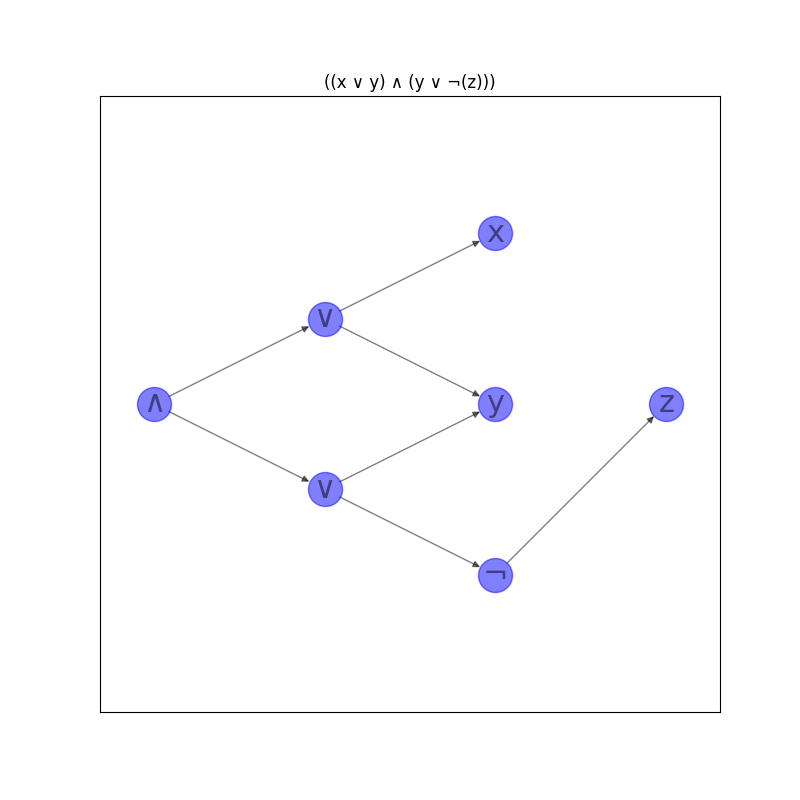# Circuits¶

Convert a Boolean circuit to an equivalent Boolean formula.

A Boolean circuit can be exponentially more expressive than an equivalent formula in the worst case, since the circuit can reuse subcircuits multiple times, whereas a formula cannot reuse subformulas more than once. Thus creating a Boolean formula from a Boolean circuit in this way may be infeasible if the circuit is large.

```import matplotlib.pyplot as plt
import networkx as nx

def circuit_to_formula(circuit):
# Convert the circuit to an equivalent formula.
formula = nx.dag_to_branching(circuit)
# Transfer the operator or variable labels for each node from the
# circuit to the formula.
for v in formula:
source = formula.nodes[v]["source"]
formula.nodes[v]["label"] = circuit.nodes[source]["label"]
return formula

def formula_to_string(formula):
def _to_string(formula, root):
# If there are no children, this is a variable node.
label = formula.nodes[root]["label"]
if not formula[root]:
return label
# Otherwise, this is an operator.
children = formula[root]
# If one child, the label must be a NOT operator.
if len(children) == 1:
child = nx.utils.arbitrary_element(children)
return f"{label}({_to_string(formula, child)})"
# NB "left" and "right" here are a little misleading: there is
# no order on the children of a node. That's okay because the
# Boolean AND and OR operators are symmetric. It just means that
# the order of the operands cannot be predicted and hence the
# function does not necessarily behave the same way on every
# invocation.
left, right = formula[root]
left_subformula = _to_string(formula, left)
right_subformula = _to_string(formula, right)
return f"({left_subformula} {label} {right_subformula})"

root = next(v for v, d in formula.in_degree() if d == 0)
return _to_string(formula, root)
```

## Create an example Boolean circuit.¶

This circuit has a ∧ at the output and two ∨s at the next layer. The third layer has a variable x that appears in the left ∨, a variable y that appears in both the left and right ∨s, and a negation for the variable z that appears as the sole node in the fourth layer.

```circuit = nx.DiGraph()
# Layer 0
# Layer 1
# Layer 2
# Layer 3
# Convert the circuit to an equivalent formula.
formula = circuit_to_formula(circuit)
print(formula_to_string(formula))

labels = nx.get_node_attributes(circuit, "label")
options = {
"node_size": 600,
"alpha": 0.5,
"node_color": "blue",
"labels": labels,
"font_size": 22,
}
plt.figure(figsize=(8, 8))
pos = nx.multipartite_layout(circuit, subset_key="layer")
nx.draw_networkx(circuit, pos, **options)
plt.title(formula_to_string(formula))
plt.axis("equal")
plt.show()
```Out:

```((x ∨ y) ∧ (y ∨ ¬(z)))
```

Total running time of the script: ( 0 minutes 0.136 seconds)

Gallery generated by Sphinx-Gallery